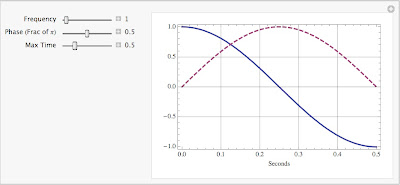## Tuesday, October 7, 2008

### Phase and time

The issue came up the other day about phase and apparent time shift.  A certain reflection coefficient was found to be complex and have a phase that is a linear function of frequency.  Numerical experiments showed a curious behavior; each frequency component was time shifted equally.  When all the frequencies were added up the resulting waveform was zero phase, even though the phase was linear with frequency.

This goes back to a Seismos column from May 2002 about the various interpretations of the word 'phase'.  The meaning at issue here is the apparent time shift associated with a particular phase at a given frequency.  Think about a cosine with zero phase.  Now let the phase be pi/2 and the cosine becomes a sine, whose peak coincides with the zero crossing of the original cosine.  By definition this zero crossing occurs at 1/4 of the period of the cosine.  If the phase is pi then the first peak lines up with the first trough of the original cosine, for an apparent time shift of 1/2 of the period.  Letting the period of the wave be T=1/f, where f is the frequency in Hz, we can summarize this as follows:
• phase=0              t-shift=0
• phase=pi/2          t-shift=T/4
• phase=pi              t-shift=T/2
Or as an equation:  t-shift = phase * T / (2 pi) = phase / (2 pi f)

In other words, if the phase is a linear function of frequency then the apparent time shift will be the same for all frequencies (because the f cancels out).  This is in agreement with the numerical tests that showed the summation over all frequencies generated a zero phase, t-shifted waveform.

The figure below is a Mathematica plot that illustrates the connection between phase and apparent time shift for a single frequency.  The solid curve is zero phase and the dashed curve is phase shifted.  In this case the frequency is 1 Hz, the period is 1 second, and we see that a phase shift of pi/2 moves the peak 0.25 s or one-quarter of the period in accord with our formula.